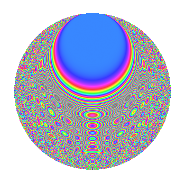# Properties

 Label 1575.2.bfLevel 1575 Weight 2 Character orbit bf Rep. character $$\chi_{1575}(551,\cdot)$$ Character field $$\Q(\zeta_{6})$$ Dimension 292 Sturm bound 480

# Learn more about

## Defining parameters

 Level: $$N$$ = $$1575 = 3^{2} \cdot 5^{2} \cdot 7$$ Weight: $$k$$ = $$2$$ Character orbit: $$[\chi]$$ = 1575.bf (of order $$6$$ and degree $$2$$) Character conductor: $$\operatorname{cond}(\chi)$$ = $$63$$ Character field: $$\Q(\zeta_{6})$$ Sturm bound: $$480$$

## Dimensions

The following table gives the dimensions of various subspaces of $$M_{2}(1575, [\chi])$$.

Total New Old
Modular forms 504 316 188
Cusp forms 456 292 164
Eisenstein series 48 24 24

## Trace form

 $$292q$$ $$\mathstrut +\mathstrut 3q^{2}$$ $$\mathstrut +\mathstrut 3q^{3}$$ $$\mathstrut +\mathstrut 139q^{4}$$ $$\mathstrut +\mathstrut 6q^{6}$$ $$\mathstrut -\mathstrut 3q^{9}$$ $$\mathstrut +\mathstrut O(q^{10})$$ $$292q$$ $$\mathstrut +\mathstrut 3q^{2}$$ $$\mathstrut +\mathstrut 3q^{3}$$ $$\mathstrut +\mathstrut 139q^{4}$$ $$\mathstrut +\mathstrut 6q^{6}$$ $$\mathstrut -\mathstrut 3q^{9}$$ $$\mathstrut +\mathstrut 3q^{12}$$ $$\mathstrut +\mathstrut 3q^{13}$$ $$\mathstrut -\mathstrut 3q^{14}$$ $$\mathstrut -\mathstrut 123q^{16}$$ $$\mathstrut +\mathstrut 9q^{17}$$ $$\mathstrut -\mathstrut q^{18}$$ $$\mathstrut +\mathstrut 6q^{19}$$ $$\mathstrut -\mathstrut 18q^{21}$$ $$\mathstrut -\mathstrut 2q^{22}$$ $$\mathstrut +\mathstrut 12q^{24}$$ $$\mathstrut +\mathstrut 18q^{26}$$ $$\mathstrut -\mathstrut 9q^{27}$$ $$\mathstrut +\mathstrut 10q^{28}$$ $$\mathstrut +\mathstrut 24q^{29}$$ $$\mathstrut -\mathstrut 21q^{31}$$ $$\mathstrut -\mathstrut 39q^{32}$$ $$\mathstrut +\mathstrut 30q^{33}$$ $$\mathstrut -\mathstrut 18q^{34}$$ $$\mathstrut -\mathstrut 60q^{36}$$ $$\mathstrut +\mathstrut 3q^{37}$$ $$\mathstrut +\mathstrut 66q^{38}$$ $$\mathstrut -\mathstrut 18q^{39}$$ $$\mathstrut +\mathstrut 6q^{41}$$ $$\mathstrut +\mathstrut 21q^{42}$$ $$\mathstrut +\mathstrut 6q^{43}$$ $$\mathstrut +\mathstrut 15q^{44}$$ $$\mathstrut -\mathstrut 4q^{46}$$ $$\mathstrut +\mathstrut 21q^{47}$$ $$\mathstrut -\mathstrut 45q^{48}$$ $$\mathstrut +\mathstrut 14q^{49}$$ $$\mathstrut -\mathstrut 18q^{51}$$ $$\mathstrut -\mathstrut 24q^{53}$$ $$\mathstrut -\mathstrut 42q^{54}$$ $$\mathstrut -\mathstrut 18q^{56}$$ $$\mathstrut +\mathstrut 21q^{57}$$ $$\mathstrut -\mathstrut 2q^{58}$$ $$\mathstrut -\mathstrut 12q^{59}$$ $$\mathstrut -\mathstrut 6q^{61}$$ $$\mathstrut -\mathstrut 24q^{62}$$ $$\mathstrut -\mathstrut 20q^{63}$$ $$\mathstrut -\mathstrut 180q^{64}$$ $$\mathstrut +\mathstrut 45q^{66}$$ $$\mathstrut -\mathstrut 8q^{67}$$ $$\mathstrut +\mathstrut 108q^{68}$$ $$\mathstrut +\mathstrut 48q^{69}$$ $$\mathstrut +\mathstrut 49q^{72}$$ $$\mathstrut +\mathstrut 6q^{73}$$ $$\mathstrut +\mathstrut 48q^{76}$$ $$\mathstrut +\mathstrut 6q^{77}$$ $$\mathstrut +\mathstrut 9q^{78}$$ $$\mathstrut -\mathstrut 14q^{79}$$ $$\mathstrut +\mathstrut 21q^{81}$$ $$\mathstrut -\mathstrut 30q^{83}$$ $$\mathstrut -\mathstrut 69q^{84}$$ $$\mathstrut +\mathstrut 63q^{87}$$ $$\mathstrut -\mathstrut 22q^{88}$$ $$\mathstrut -\mathstrut 27q^{89}$$ $$\mathstrut +\mathstrut 5q^{91}$$ $$\mathstrut -\mathstrut 42q^{92}$$ $$\mathstrut +\mathstrut 48q^{93}$$ $$\mathstrut +\mathstrut 3q^{94}$$ $$\mathstrut -\mathstrut 126q^{96}$$ $$\mathstrut +\mathstrut 3q^{97}$$ $$\mathstrut +\mathstrut 33q^{98}$$ $$\mathstrut -\mathstrut 6q^{99}$$ $$\mathstrut +\mathstrut O(q^{100})$$

## Decomposition of $$S_{2}^{\mathrm{new}}(1575, [\chi])$$ into irreducible Hecke orbits

The newforms in this space have not yet been added to the LMFDB.

## Decomposition of $$S_{2}^{\mathrm{old}}(1575, [\chi])$$ into lower level spaces

$$S_{2}^{\mathrm{old}}(1575, [\chi]) \cong$$ $$S_{2}^{\mathrm{new}}(63, [\chi])$$$$^{\oplus 3}$$$$\oplus$$$$S_{2}^{\mathrm{new}}(315, [\chi])$$$$^{\oplus 2}$$